Rules of Thermometers

## Rules of Thermometers

### Rules of Termometers

At the beginning you have a grid filled with many empty thermometers your goal is to fill the thermometers with mercury, until that at the end each column and row have  quantity of mercury indicated by the numbers outside the grid. A thermometer must be filled from the base (round part) to the top. This is an example of a puzzle and solution.### Some tips

First scan each row and column one after the other trying to put the mercury (in shading the parts of the thermometer) or to eliminate cells (marking the cell with X). Then, with this information, do again the cycle until the final resolution. This puzzle could require a lot of thinking to resolve for the most difficult (3 stars) but it is important to know the two basic technics.

### Overflowing

When the number of mercury is less than the capacity of a thermometer then the over capacity could be eliminated. By example (taken from the grid showed) in the last row the clue is 2 and a thermometer in this row has a capacity of 4.Therefore, the last two cells of the thermometer could be eliminated.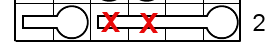### Backflow

This is when a part of a thermometer must be filled. This could happen when in a row or column have a thermometer in the direction (horizontal thermometer for row and vertical for column) and the quantity of mercury is greater than the other part of the remaining thermometers. By example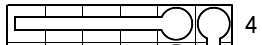this row have one big thermometer of size 5 and a part of another thermometer, the quantity of mercury for this row is 4. We could deduct that in any way the big themometer must have, at the minimum, three degrees of mercury because if the cell of the verfical mercury is filled then the big thermometer must have 3 cells filled or if the vertical thermometer is empty then the big themometer has 4 cells filled in any case the big thermometer have 3 cells filled.

Ok you have enough technics to resolve easy puzzles (2 stars and less) for the others (3 stars) you need another technic that consist to eliminate impossible cells, which will be explained later.

This puzzle comes in many sizes: 6x6 up to 11x11.

Try this captivating puzzles.

## ADVANCED TECHNIQUES FOR SOLVING THERMOMETER.

For solving some puzzles we need more advanced techniques  like the forced move and the grouping (this the names I call it). To show you how to apply these techniques I will use a puzzle sent to me by a reader. But first a short description of each strategy.

### Forced Move

This technique helps to determine if a cell is empty or full by choosing a cell such that if that cell is filled or empty then the consequence will cause contradiction. It is like calculating moves ahead iat chess . To to be efficient it requires to choose the cell that will give the biggest impact else this tecnhique is a simple trial and error method. By example if a thermometer of size 5 is empty and you choose to fill the base then nothing more else would happen but if you fill the cell at the top of the termometer then automatically all the other cells of the thermometer will be filled and it is even better if the row(or column) is by the way filled because all the other cell of the column will be automaticaly emptied. So by only one move this cause a grand impact. I distinguish different applications.

1. Elimination of filled cell.  If a cell is empty and the resulting situation causes a contradiction then this cell must be emptied.

2. Elimination of empty cell. If a cell is empty and the resulting situation causes a contradiction then this cell must be filled. Again we must choose a cell  that will give the bigger impact. By example, for an empty thermometer of size 5,  we must empty the base then automaticly all the other 4 cells of the thermometer will be emptied.

### Grouping

On some occasion it could be useful to group columns or rows together. By example if a row has a capacity of 2 degrees of mercury and only 3 cells are free from 3 different thermometers we cannot decide how to put mercury. But if we notice that 2 thermometers cannot have together mercury( they are mutually exclusive) then we can regroup these two thermometers and count them as ,1 so the cell of the third thermometer will have the mercury. The resolution of the example e will show you the 2 techniques.

## Example

This is the puzzle (a picture of the puzzle more precisely), that a reader submit me, after using the basic strategies he was not able to progress more.(see  figure 1).Figure 1

For more clarity, I assign a letter for each column (A to I) and a number for each row (1 to 12). So for applyiing the method of forced move, we must pick up a cell giving the biggest impact if filled or emptied, so the cell F5 seems to be perfect for that. This cell is at the top of a themometer of size 5 and if the thermometer is full then by the way the column F will be full and also the row 5 will be full. I use the color yellow to show the impacts of fillling F5 ( see figure 2).Figure 2

The result (in yellow) is forced by filling F5, the themometer is full, the column F is completed, the row 2 and 5 are completed. The row 4 has three degrees to allocate and only two cells available, this is a contradiction and we could conclude that F5 is empty (see figure 3). We have progressed a little but lets continue.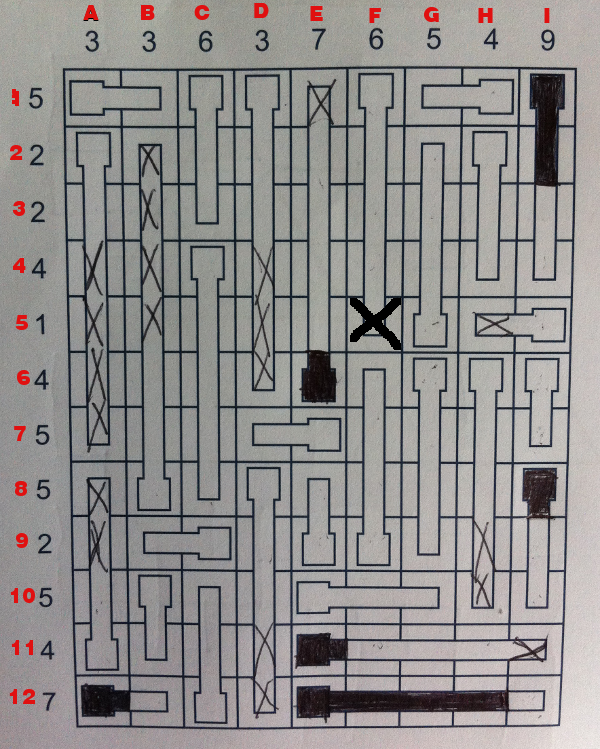Figure 3

The row 5 is a good row to apply the technique of the forced move because it has a capacity of one degree, so filling a cell  imply to empty all the other cells of the row. So if we choose C5 to fill, the following situation arises (figure 4).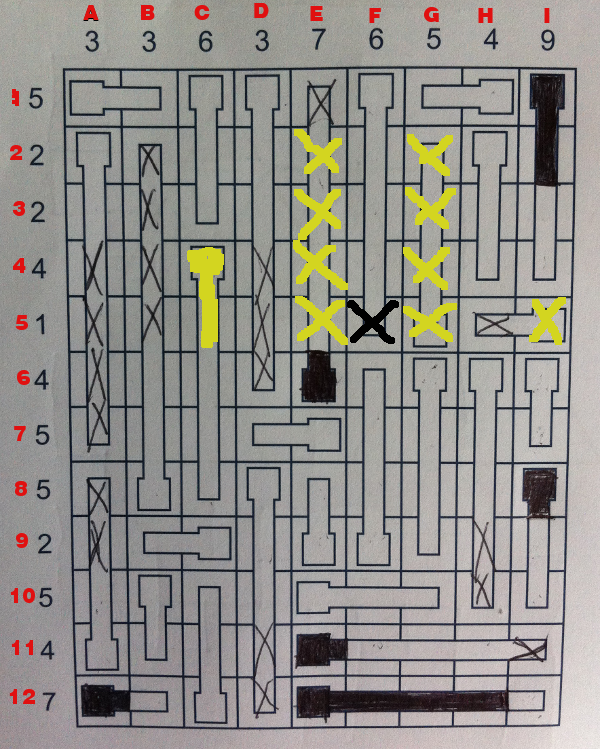Figure 4

The row 4 has 3 degrees to allocate and they are 3 free cells in this row, it seems at first that we find a perfect match but by calculating (again) a move ahead, we notice that the three different cells will be filled (F4,H4 ,I4) and also by the same way the cells (F3,h3 and I3) will be filled then the capacity of the row 3 will be exceeded, because the row 3 could only accept 2 degrees and now the row 3 has 3 degrees. So C5 is empty and  by the way C6,C7 et C8  are empty (see figure 5).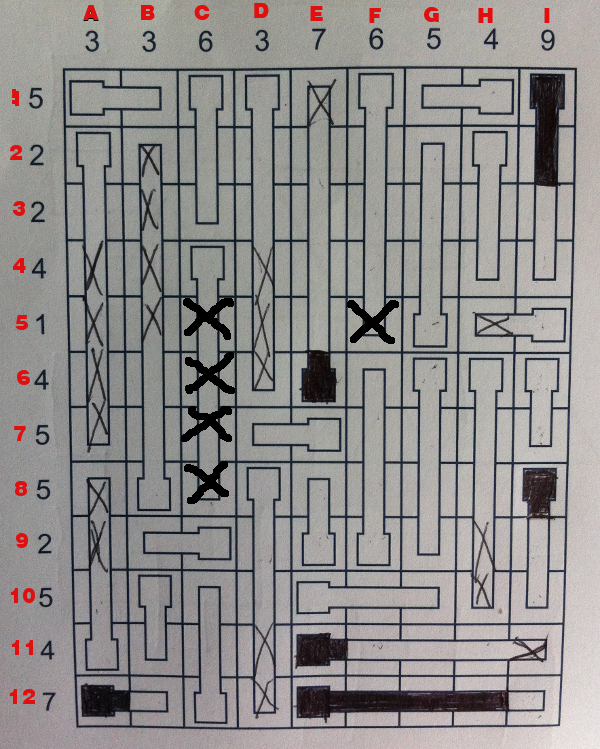Figure 5

If we try I5 then the following result appears (figure 6).Figure 6

So the 4 free cells of the row 4 will be filled and agains the row 3 will have 3 cells filled (F4,H4 and I4) instead of 2. So we eliminate I5 (figure 7).Figure 7

## Grouping (application)

We have noticed something special from the preceding moves. First that the rows 3,4 and 5 are linked together. The row 4 has 4 degrees left for 6 free cells, we know that the cells E4 and G4 cannot have mercury together because the row 5 will exceed ( the row will have 2 degrees for a capaciity of 1) . So these 2 cells (E4 and G4) can only receive 1 degree then we could group these two cells together. If the 3 cells  F4,H4 et I4 were filled then the row 3 will be exceeded because if the cells F4,H4 and I4 are filled then the cells F3,H3 and I3 will also be filled and this will result that the row 3 will have 3 degrees instead of 2. So we could form a group with F4,H4 and I4 with a capacity at most of 2 degrees. We now have first group of 2 cells with a capacity of 1 degree, and a second group of 3 cells with a capacity of 2 degrees. Then it remains only one cells in this row with the capacity of 1 and it is the cell C4. So by making group we are able to attribute 1 degree to C4 (figure 8).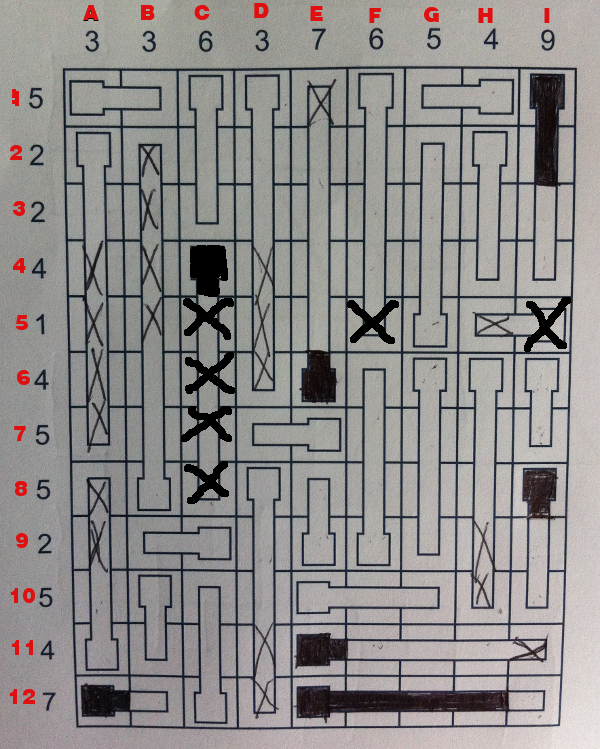Figure 8

The  column I with 6 degrees left to attribute for only 7 cells let us to use basic techniques to fill  I3,I6 et I9 (figure 9).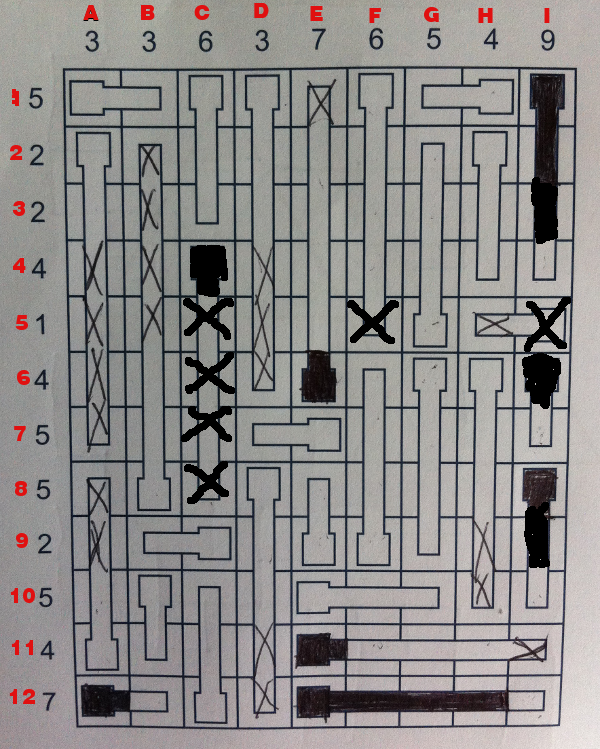Figure 9

We eliminate B9 by overflow . And by the grouping method I9 is filled because in row 4 it left 3 degrees and we could form a group with E4 and G4 with a capacity of 1 degree and a second group with F4 and H4 with a capacity of 1 degree so the cell outside of these two groups, I4 must receive 1 degree ( figure 10).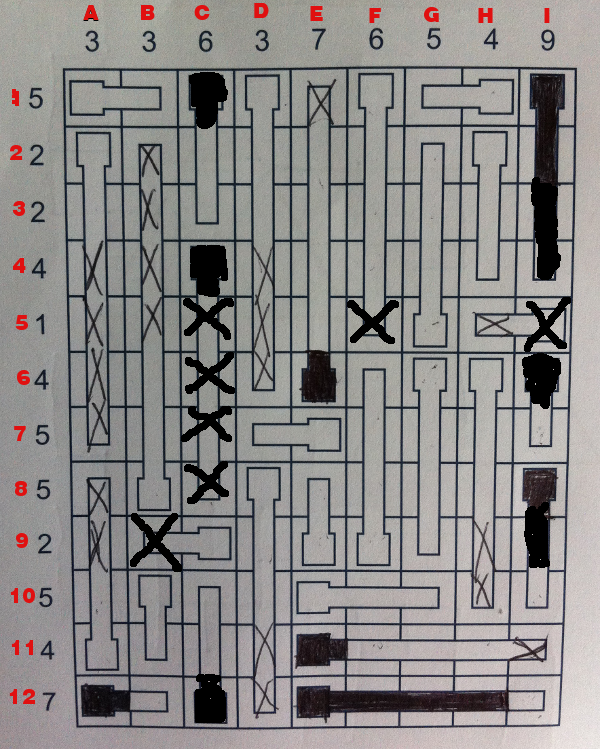Figure 10

So i was able to finish the puzzle with the sames techniques ( I retried many times because I went to fast ) .Figure11

Voila , like many way lead to Rome, it has many ways to resolve thermometer. You will discover your own techniques.If so I will be pleased to receive your comments.

Comments powered by CComment